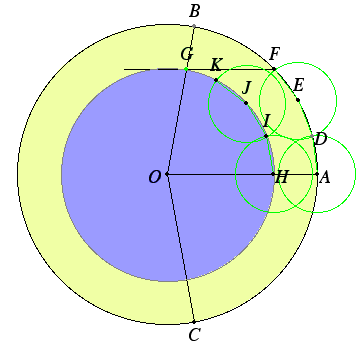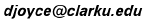### Trisect?

Another idea for trisecting an angle. Take an arbritrary angle AOB and place it in a circle with center at O.

Extend lines, bisect angles, connect points, etc., to get this figure.Now extend CK to meet the circle at N, and extend DL to meet the circle at N, and draw some more lines.Claim: the angle AOB is trisected by OM and ON. That is, the three angles AON, NOM, and MOB are equal. They certainly appear to be equal, even for angles near 180 degrees.

The proposed proof starts with the claim that angle NDA equals angle MOF. The rest of the proof follows from that statement. By Euclid's III.20, "In a circle the angle at the center is double the angle at the circumference when the angles have the same circumference as base," angle NDA is half of angle NOA. By symmetry, you can see that angle MOF is half of angle MON. Therefore, NOA = MON, and, of course, by symmetry the two angles NOA and MOB are equal, so angle AOB is trisected.

Note that two sides of the angles NDA and MOF are parallel, namely, side DA is parallel to side OF. Thus, the claim that the two angles are equal is logically equivalent to the statement that the other two sides, namely, side DN and side OM, are parallel. They look like they're parallel in the figure. You can see that they're not, though, when the original angle AOB is very close to 180 degrees.

Thus, this is not an angle trisection. It's close enough, though, so that it fools the eye.

It was proved in the 19th century that angles cannot be trisected with the Euclidean tools of straightedge and compass.